# Probability distribution functions Normal distribution Lognormal distribution Mean

• Slides: 14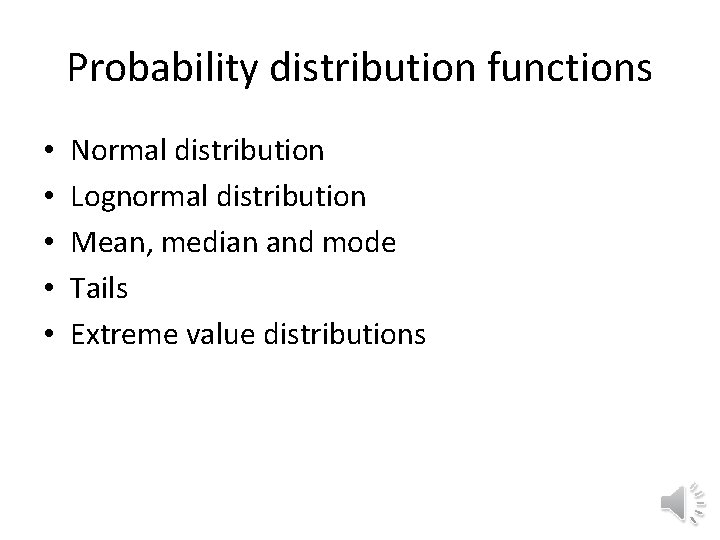Probability distribution functions • • • Normal distribution Lognormal distribution Mean, median and mode Tails Extreme value distributionsNormal (Gaussian) distribution • Probability density function (PDF) • What does figure tell about the cumulative distribution function (CDF)?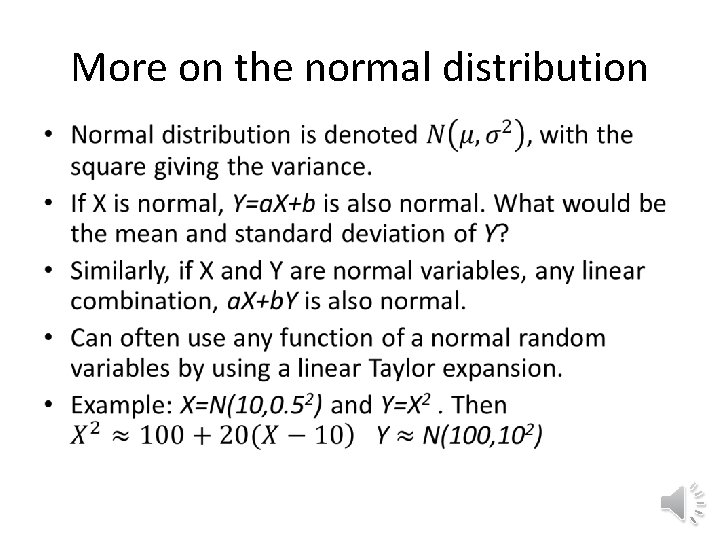More on the normal distribution •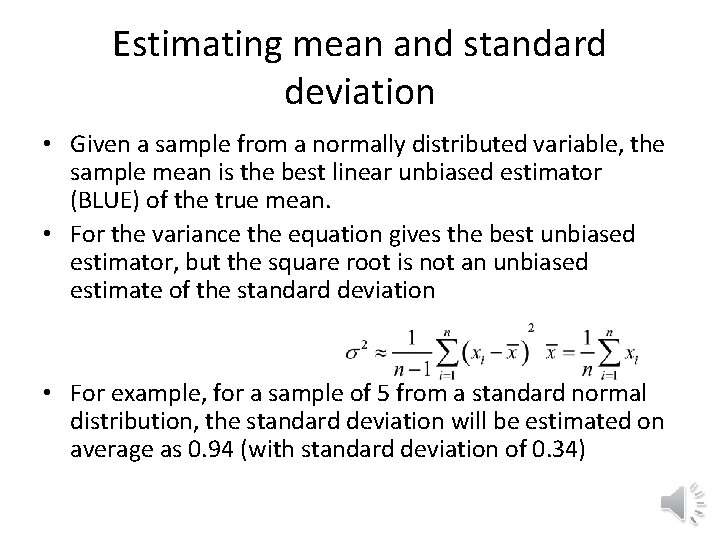Estimating mean and standard deviation • Given a sample from a normally distributed variable, the sample mean is the best linear unbiased estimator (BLUE) of the true mean. • For the variance the equation gives the best unbiased estimator, but the square root is not an unbiased estimate of the standard deviation • For example, for a sample of 5 from a standard normal distribution, the standard deviation will be estimated on average as 0. 94 (with standard deviation of 0. 34)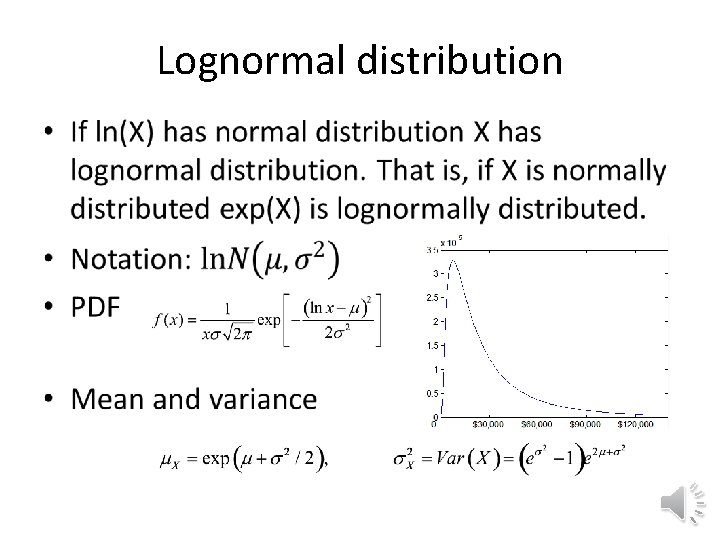Lognormal distribution •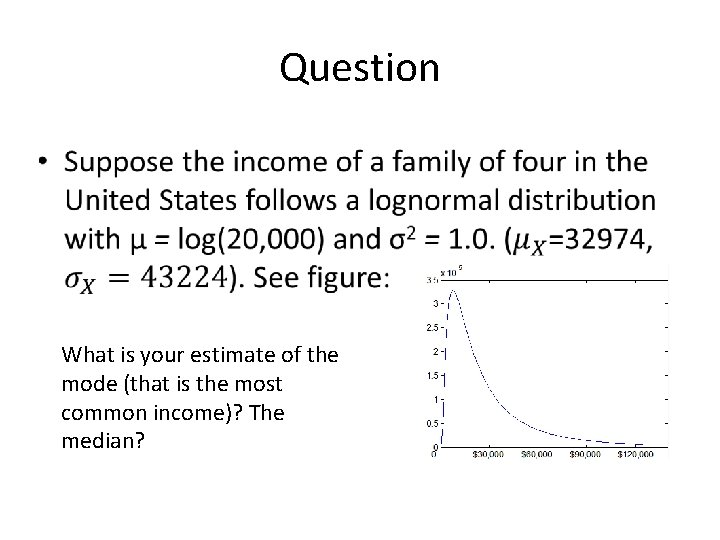Question • What is your estimate of the mode (that is the most common income)? The median?Mean, mode and median •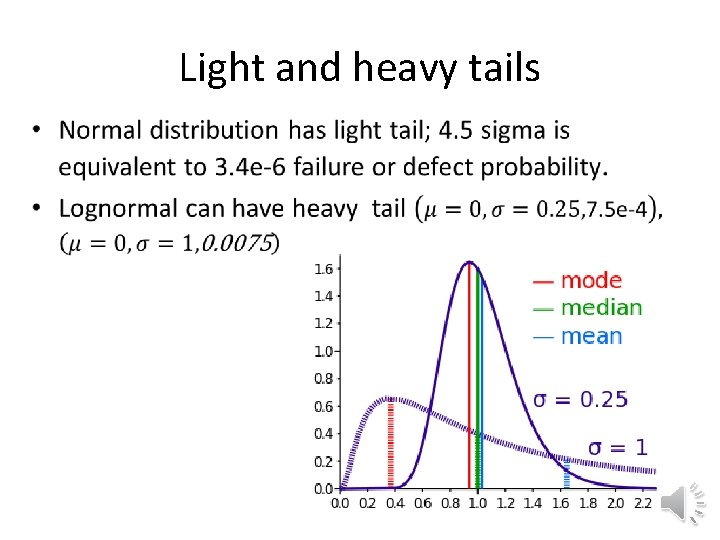Light and heavy tails •Fitting distribution to data • Usually fit CDF to minimize maximum distance (Kolmogorov-Smirnoff test) • Generated 20 points from N(3, 12). • Normal fit N(3. 48, 0. 932) • Lognormal ln. N(1. 24, 0. 26) Almost same mean and standard deviation.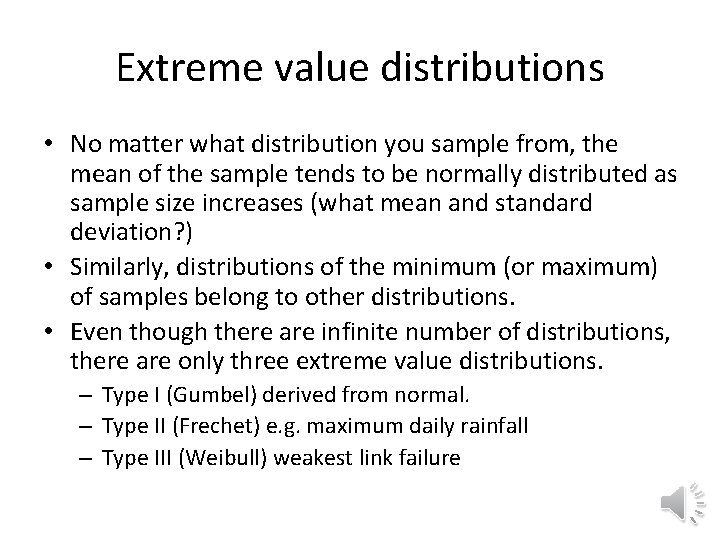Extreme value distributions • No matter what distribution you sample from, the mean of the sample tends to be normally distributed as sample size increases (what mean and standard deviation? ) • Similarly, distributions of the minimum (or maximum) of samples belong to other distributions. • Even though there are infinite number of distributions, there are only three extreme value distributions. – Type I (Gumbel) derived from normal. – Type II (Frechet) e. g. maximum daily rainfall – Type III (Weibull) weakest link failureMaximum of normal samples With normal distribution, maximum of sample is more narrowly distributed than original distribution. Max of 10 standard normal samples. 1. 54 mean, 0. 59 standard deviation Max of 100 standard normal samples. 2. 50 mean, 0. 43 standard deviation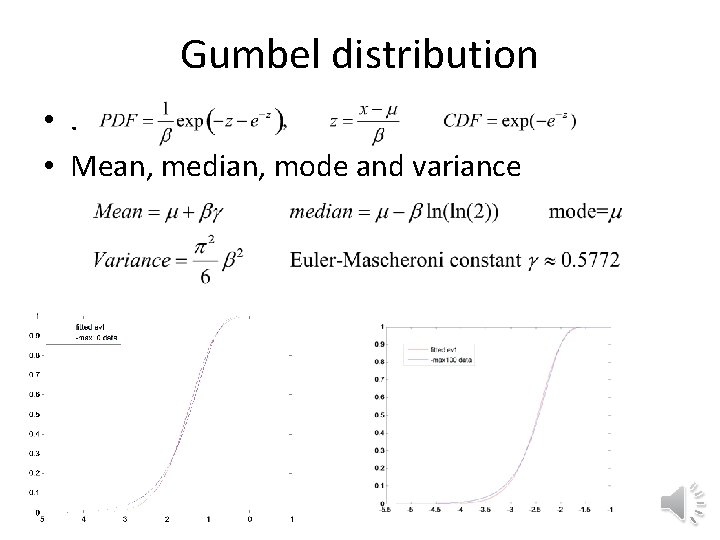Gumbel distribution • . • Mean, median, mode and varianceWeibull distribution • Probability distribution • Its log has Gumbel dist. • Used to describe distribution of strength or fatigue life in brittle materials. • If it describes time to failure, then ü k<1 indicates that failure rate decreases with time, ü k=1 indicates constant rate, ü k>1 indicates increasing rate. • Can add 3 rd parameter by replacing x by x-c.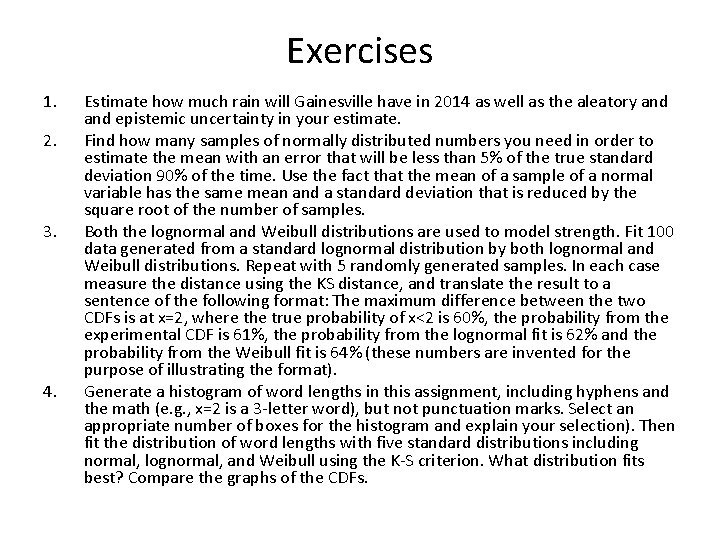Exercises 1. 2. 3. 4. Estimate how much rain will Gainesville have in 2014 as well as the aleatory and epistemic uncertainty in your estimate. Find how many samples of normally distributed numbers you need in order to estimate the mean with an error that will be less than 5% of the true standard deviation 90% of the time. Use the fact that the mean of a sample of a normal variable has the same mean and a standard deviation that is reduced by the square root of the number of samples. Both the lognormal and Weibull distributions are used to model strength. Fit 100 data generated from a standard lognormal distribution by both lognormal and Weibull distributions. Repeat with 5 randomly generated samples. In each case measure the distance using the KS distance, and translate the result to a sentence of the following format: The maximum difference between the two CDFs is at x=2, where the true probability of x<2 is 60%, the probability from the experimental CDF is 61%, the probability from the lognormal fit is 62% and the probability from the Weibull fit is 64% (these numbers are invented for the purpose of illustrating the format). Generate a histogram of word lengths in this assignment, including hyphens and the math (e. g. , x=2 is a 3 -letter word), but not punctuation marks. Select an appropriate number of boxes for the histogram and explain your selection). Then fit the distribution of word lengths with five standard distributions including normal, lognormal, and Weibull using the K-S criterion. What distribution fits best? Compare the graphs of the CDFs.